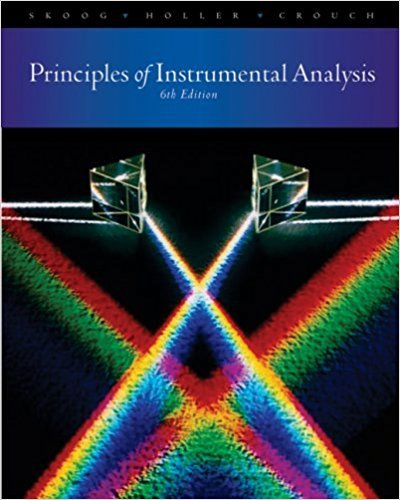> > > > Problem 10-11

# The chromium in a series of steel samples was determined by fCP emissionspectroscopy## Problem 10-11 Chapter 10

Principles of Instrumental Analysis | 6th Edition

• 2901 Step-by-step solutions solved by professors and subject experts
• Get 24/7 help from StudySoup virtual teaching assistantsPrinciples of Instrumental Analysis | 6th Edition

4 5 0 388 Reviews
18
4
Problem 10-11

The chromium in a series of steel samples was determined by fCP emissionspectroscopy. The spectrometer was calibrated with a series of standardscontaining O.2.0, -l.O,6.0. and 8.0 /lg K,Cr,O, per milliliter. The instrument readings for these solutions were 3.1, 21.5, 40.9, 57.1, and 77.3. respectively, inarbitrary units.(a) Plot the data using a spreadsheet.(b) Find the equation for the regression line.(c) Calculate standard deviations for the slope and the intercept of the line in (b).(d) The following data were obtained for replicate 1.00-g samples of cementdissolved in HCI and diluted to 100.0 mL after neutralization.Calculate the percentage of Cr,03 in each sample. What are the absoluteand relative standard deviations for the average of each determination?

Step-by-Step Solution:
Step 1 of 3

I. Chapter 11 Continued A. Concentration Units 1. Molarity (M) a. M = moles/liters b. [KCl] means molarity of a KCl solution 2. Molality (m) a. m = moles/kg of solvent b. mass-based and independent of temperature 3. Mass Fraction a. Mass fraction = mass...

Step 2 of 3

Step 3 of 3

##### ISBN: 9780495012016

Unlock Textbook Solution

The chromium in a series of steel samples was determined by fCP emissionspectroscopy

×
Get Full Access to Principles Of Instrumental Analysis - 6 Edition - Chapter 10 - Problem 10-11

Get Full Access to Principles Of Instrumental Analysis - 6 Edition - Chapter 10 - Problem 10-11

I don't want to reset my password

Need help? Contact support

Need an Account? Is not associated with an account
We're here to help